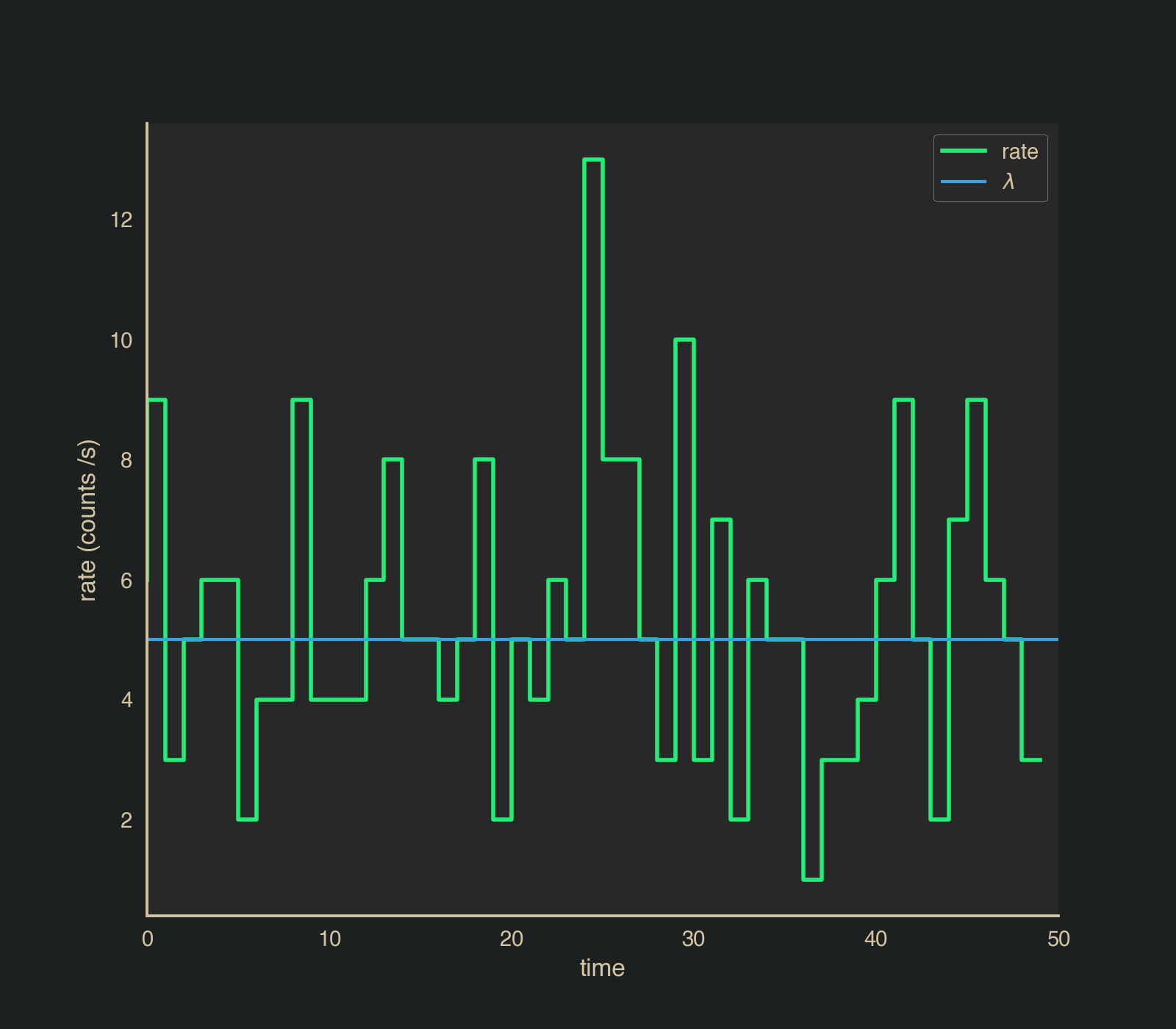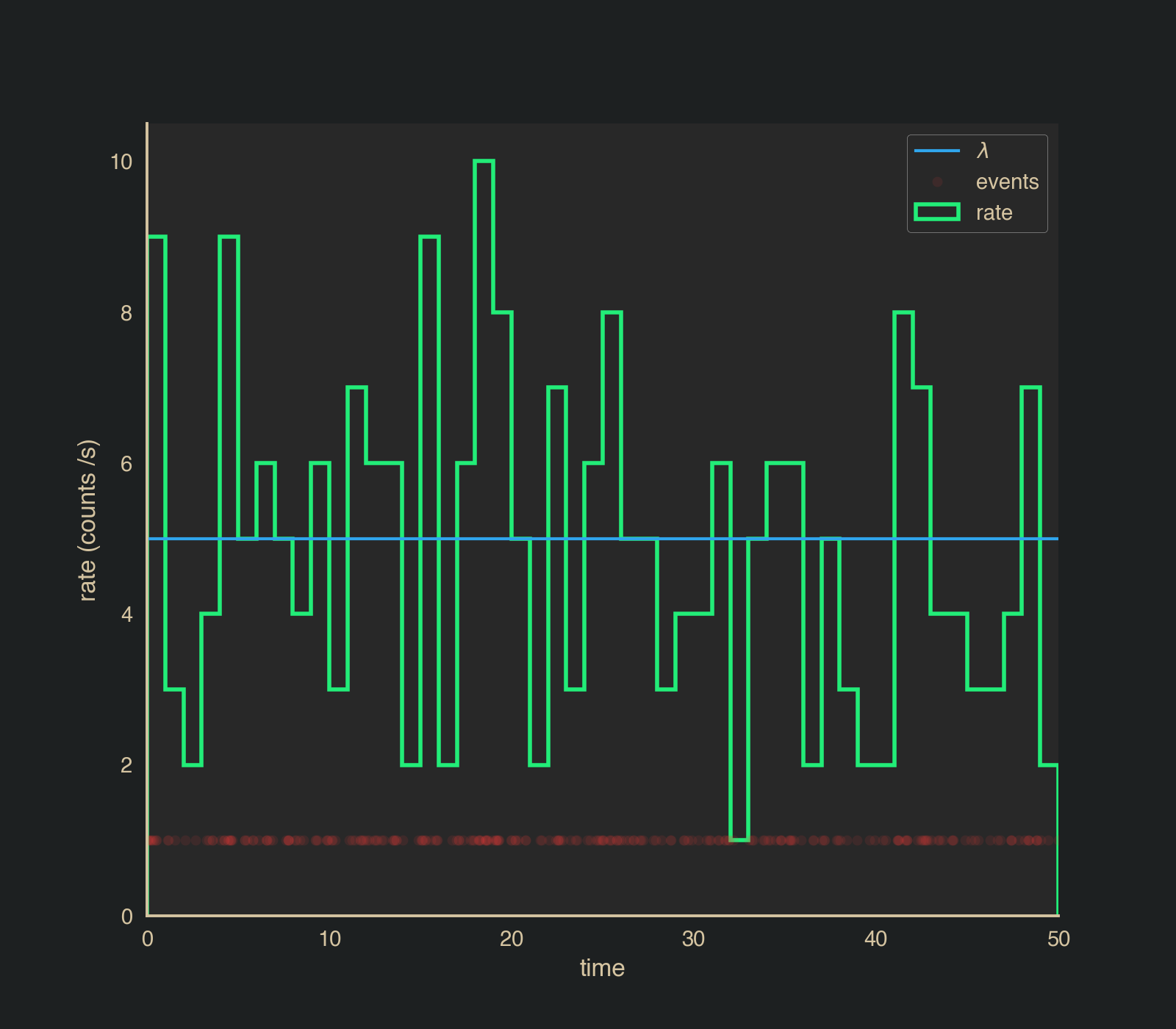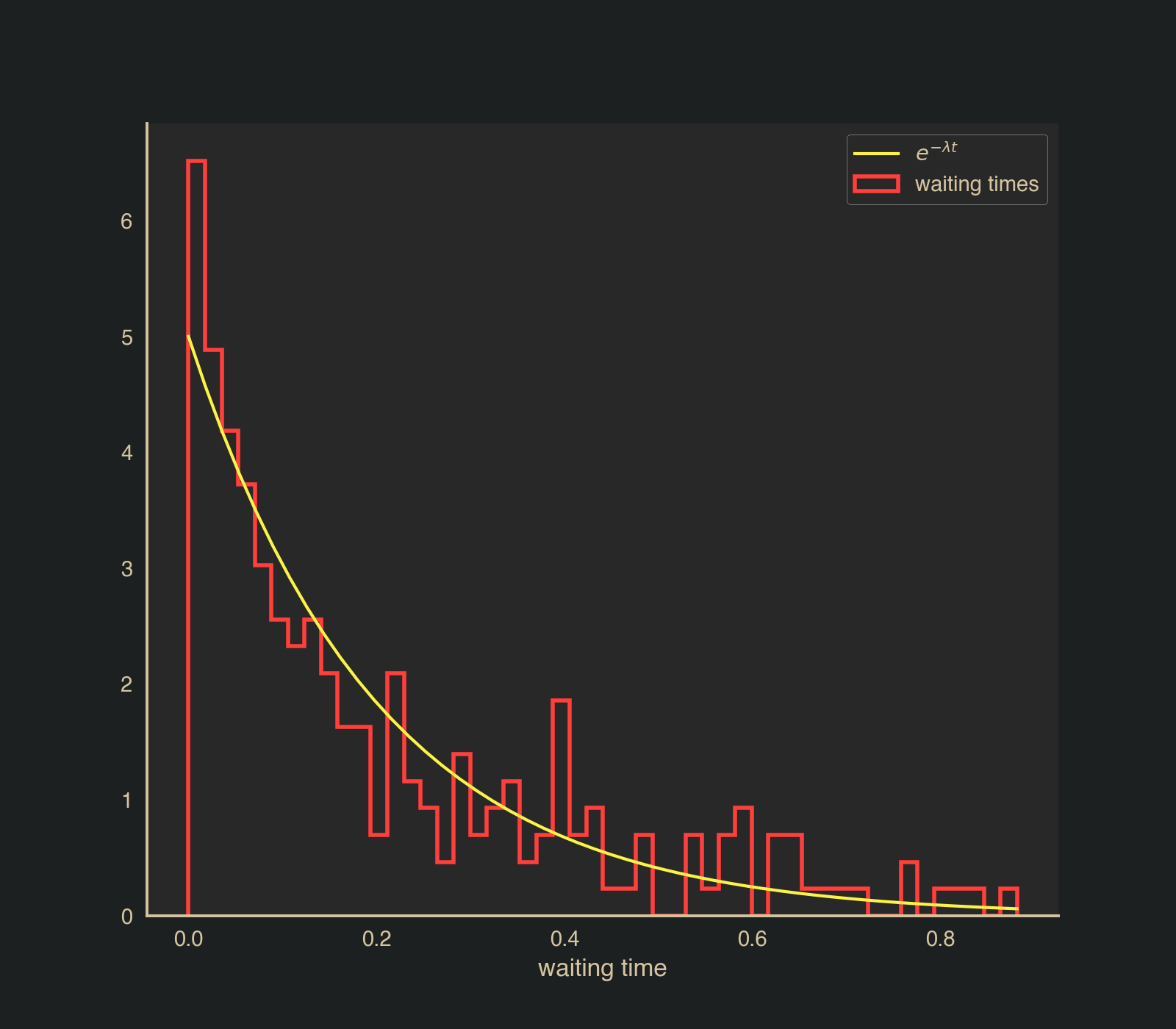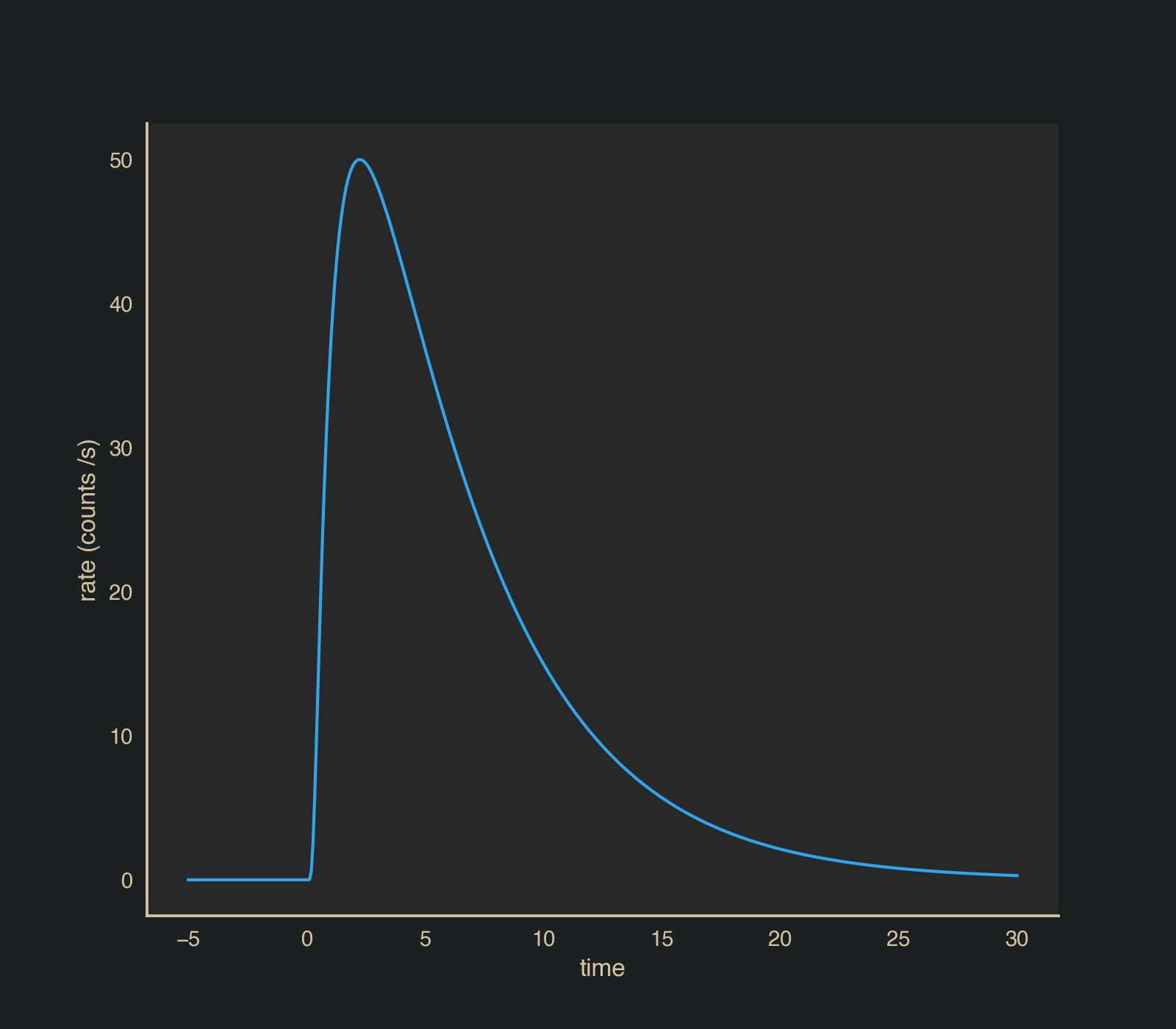Generating synthetic Poisson time series

Updated:

In x-ray astronomy, we often encounter Poisson distributed time series. This leads to lots of different time series analysis approaches. Whenever we want to try a new analysis, it is a good idea to first work on a simulated data set so that we can control the experiment. The goal will be to generate individual arrival times such that the events have the proper distribution, i.e., that they know about each other.

%matplotlib notebook
import matplotlib.pyplot as plt
from jupyterthemes import jtplot
plt.style.use('mike')
jtplot.style(context='notebook', fscale=1, grid=False)

red = '#FF3F3C'
green = '#22ED78'
yellow =  '#FBF346'
blue = '#30A5EC'

np.random.seed(1234)

The naive way

First lets look at a very simple way to create a light curve. We know counts are Poisson distributed. Let’s say we want to generate a constant light curve with a rate $$\lambda=5$$ counts per second. We could choose time bins of one second duration, and draw from the Poisson distribution.

dt = 1.
starts = np.arange(0, 50, dt)

rate = 5.

fig, ax = plt.subplots()

y = np.random.poisson(rate, size=len(starts))

ax.step(starts,y,color=green, label = 'rate', lw=2)

ax.axhline(rate, label=r'$\lambda$',color=blue)
ax.set_xlabel('time')
ax.set_ylabel('rate (counts /s)')
ax.set_ylabel('rate (counts /s)')
ax.set_xlim(0,50)
ax.legend()

<IPython.core.display.Javascript object><matplotlib.legend.Legend at 0x1096dc710>

We have a light curve and it exhibits the expected Poisson variability we expect, but we are stuck here. We cannot do very much with the counts if, for example, we would like to rebin or add properties to individual events… because we do not have events at the moment. Let’s see how we can do better.

A constant rate

First we will explore a constant rate. What we want to generate are arrival times of events such that the events are Poisson distributed.

$\pi(f(t) = n | \lambda)=\frac{\lambda t ^{n} e^{-\lambda y }}{n !}$

which tells us the probability of observing $$n$$ events over a time from now until $$t$$ given a rate of events $$\lambda$$.

If we want to know how long we must wait, $$T$$, until a new event arrives, we have are asking the question:

$\pi(T>t) = \pi(f(t)=0) = \frac{(\lambda t)^0 e^{-\lambda t}}{0!} = e^{-\lambda t}$

Thus, the waiting time is distributed exponentially and if we want to sample events arriving at these times we need to sample this distribution. We can achieve this via inverse transform sampling.

The cumulative distribution function for the exponential distribution is

$$F(t) = 1 - e^{-\lambda t}$$.

When thus need to solve

$F(F^{-1}(u)) = u$

where $$u \sim U(0,1)$$. This yields

$t = F^{-1}(u) = -\frac{1}{\lambda} \log(1-u)$

and since the shape of the distribution does not change by shifting $$u$$, we can simple have

$$t = F^{-1}(u) = -\frac{1}{\lambda} \log(u)$$.

Now this tells us how to draw a new waiting time which is a duration. So if we want to draw a time series, we simply need to start from a time $$t_0$$, draw a waiting time, and then add this to $$t_0$$. We can repeat this until we reach the time we desire. Let’s try it out.

# this is our t0
current_time = 0
# this is the time we would like to reach
max_time = 50.

# this is our lambda
rate = 5. # evts/s

# we store the arrival times and we start with our current time
arrival_times = [current_time]

# we stop when we exceed our current time
while current_time < max_time:

# draw a uniform random number
u = np.random.uniform(0,1)

# compute a new time by adding the waiting time to
# the current time
new_time = current_time - (1./rate) * np.log(u)

# store this new time
arrival_times.append(new_time)

# update the current time
current_time = new_time

Now let’s look and our binned light curve. We bin such that each bin is one second long.

fig, ax = plt.subplots()

bins = np.arange(0,51, 1)

ax.hist(arrival_times,bins=bins, histtype='step',color=green, lw=2, label='rate');
ax.axhline(rate, label=r'$\lambda$', color=blue)
ax.plot(arrival_times, np.ones_like(arrival_times),'o', color=red, label='events',markersize=5,alpha=0.1)

ax.set_xlabel('time')
ax.set_ylabel('rate (counts /s)')
ax.set_xlim(0,50)
ax.legend();
<IPython.core.display.Javascript object>So we have made a light curve! We can see deviations around the mean rate which are normal Poisson fluctuations we expect and similar to what we simulated before.

However, remember that the point of this is to generate individual events and not simply a Poisson number of counts. We should examine those events, and more specifically, we need to see if the waiting time between events is actually exponentially distributed.

fig, ax = plt.subplots()

bins = np.arange(0,51, 1)

# compute the distribution of the waiting time
# between the events
dt = np.diff(arrival_times)

xx = np.linspace(0,max(dt))

ax.hist(dt,bins=50,  histtype='step',color=red, lw=2, density=True, label='waiting times');

# plot the normalized exponential distribution
ax.plot(xx, rate * np.exp(-rate*xx), label=r'$e^{-\lambda t}$', color = yellow)

ax.set_xlabel('waiting time')

ax.legend();

<IPython.core.display.Javascript object>Excellent, our procedure seems to be working. Since we have events, we can easily rebin our light curve or perform other operations on them that would not be possible if we only simulated counts in different bins. The algorithm can be made more efficient, but this demonstrates the basics of the idea.

So, a constant rate is rather boring. What if we want to simulate a changing rate?

A non-homogeneous Poisson rate

If the rate is changing with time, i.e., we have $$\frac{d \lambda(t)}{d t} \neq 0$$, then we need to do a bit more to preserve the changing Poisson properties of the light curve. first, let’s chose the function we would like our light curve to be simulated from

# I am using numba because we will draw a lot of events
from numba import jit, njit

@jit
def norris(x, K, t_start, t_rise, t_decay):
"""
This is a function often used to model gamma-ray burst
light curve from CITE HERE

x: time
K: value at maximum
t_start: when the pulse starts
t_rise: the duration of the rise
t_decay: the duration of the decay

"""
if x > t_start:
return (
K
* np.exp(2. * (t_rise / t_decay)**(0.5))
* np.exp(-t_rise / (x - t_start) - (x - t_start) / t_decay)
)
else:
return 0.0
fig, ax = plt.subplots()

lc_start = -5.
lc_stop = 30.

time = np.linspace(lc_start,lc_stop,500)

tstart = 0.
trise= 1.
tdecay = 5.
K = 50.
y = np.array([norris(t, K, tstart, trise, tdecay) for t in time])

ax.plot(time, y, color=blue, label='pulse')

ax.set_xlabel('time')
ax.set_ylabel('rate (counts /s)');

<IPython.core.display.Javascript object>Now, the trick is to keep the waiting times connected to the exponential distribution but also respect the changing rate with time. We can achieve this sampling a constant rate defined by the maximum of the pulse and then thinning or rejection sampling the events. Since the process is slightly complicated, we will define a function to do this. We will also store some extra information so that we can examine what occurs during the algorithm.

@jit(forceobj=True)
def pulse_simulator(lc_start, lc_stop, K, p_start, t_rise, t_decay):
"""
Non-homogeneous poisson process generator
for a given max rate and time range, this function
generates time tags sampled from light curve

lc_start: when to start the light curve
lc_stop: when to stop the light curve
K: the pulse maximum
p_start: the pulse start
t_rise: the duration of the rise
t_decay, the duration of the decay

"""

# if there is no pulse, return nothing
if K == 0.:
return np.empty(1)
else:

# the maximum is L
f_max = K

# set time to the beginning of the light curve
time = lc_start

# blank array for arrival times
arrival_times = np.array([],dtype=np.float64)

# only append to first photon if the pulse has started
if p_start<=lc_start:
arrival_times = np.append(arrival_times,lc_start)

# we are going to store some auxilary information
# for plotting later
tests = []
accepted = []
times = []

while time < lc_stop:

# sample from the inverted CDF assuming the
# rate is always at the maximum of the pulse
time = time - (1.0 / f_max) * np.log(np.random.rand())

# pull a uniform random number for a test
test = np.random.rand()

# compute height of the pulse at this time
# and normalize it between 0 and 1.
p_test = norris(time, K, p_start, t_rise, t_decay) / f_max

# if the random test we pulled is less
# then the value of the pulse at the current time
# we accept this time and store it
if test <= p_test:
arrival_times= np.append(arrival_times, time)
accepted.append(True)
else:
accepted.append(False)

# keep track of all times and
# tests for plotting
times.append(time)
tests.append(test)

return np.array(arrival_times), np.array(accepted), np.array(times), np.array(tests)
arrival_times, accepted, all_times, tests = pulse_simulator(lc_start=lc_start,
lc_stop=lc_stop,
K=K,
p_start=tstart,
t_rise=trise,
t_decay=tdecay)

Let’s have a look at what we simulated by plotting all the event “test” values in the normalized space along with the normalized pulse.

fig, ax = plt.subplots()

time = np.linspace(lc_start,lc_stop,500)

y = np.array([norris(t, K, tstart, trise, tdecay) for t in time])

ax.plot(time, y/K, color=blue, label='pulse')
ax.axhline(1.,color=yellow,  label='maximum')

ax.scatter(all_times[accepted],tests[accepted], facecolor=green,edgecolor='none' ,alpha=0.5, label='accepted')
ax.scatter(all_times[~accepted],tests[~accepted],facecolor=red, edgecolor='none' ,alpha=0.5, label='rejected')
ax.set_ylim(0)
ax.set_xlabel('time');
ax.set_ylabel('normalized rate (counts /s)');
ax.legend();

<IPython.core.display.Javascript object>